# Statistical Significance: Are You Interpreting Correctly?

## Know the null hypothesis!

A few days back, I was chatting with a friend about a paper. At one point, the conversation went like this:

Friend: (Referring to a result mentioned in the paper) the difference in the average outcome between the two groups is statistically significant.

I: yeah, it just suggests that the difference in the average outcome between the two groups is highly likely to be different from 0; but from this result, we get no idea on how big the actual difference is.

Friend: What!? 😲 I thought a statistically significant difference suggests a substantial difference in the outcome! 🤷‍♂️

Indeed many of us have the same misunderstanding, particularly at the beginning of our statistics education. And now that data is everywhere and statistical results are often shared with people in general — particularly in newspapers, magazines, and blogs — it is important not to misguide anyone.

Colloquially, when we say that something is significant, we refer to the meaningfulness and importance of it. If you received no education in inferential statistics or if you were just poorly instructed, intuitively, you may think that a statistically significant finding means an important finding obtained through a statistical test. Yes, a statistically significant finding may be really important in some cases, but it may not be important at all in many other cases. For example, a \$5 difference in the average annual income between two groups of people may be statistically significant (i.e. highly likely to be different from 0 in the population of interest) in a large sample, but this difference may not have any real-world significance.

Here are a few things to note about statistical significance in the context of two-tailed t-tests/z-tests:

1. Statistical significance is always tied to a hypothesis test. Usually, we have: i) a null hypothesis of no difference and ii) an alternative hypothesis of a non-zero difference in the average outcome between the two groups in the population of interest. It should be noted that the null hypothesis can include any number, not just 0. For example, in a two-sample t-test, we can test the null hypothesis of a \$1000 difference (rather than a \$0 difference) in the population-level average annual income between the two groups. In many statistical packages, the default value of the difference in the null hypothesis is set to 0. This makes sense because most of the time we test a null hypothesis of no difference in the population-level average outcome between two groups.
2. We decide on a significance level (expressed by the Greek letter alpha) for the hypothesis test. Conventionally, we pick a 5% significance level which means we expect to make a correct decision on the hypothesis test 95 out of 100 times (conversely, this implies: we take a risk of erroneously rejecting a true null hypothesis 5 out of 100 times). Based on the significance level and the p-value (probability of getting a sample estimate of the difference in the average outcome, identical to or more extreme than the one that we got in our sample, given the null hypothesis is true) of the hypothesis test, we set a decision rule: if the p-value is ≤0.05, we reject the null hypothesis in favor of the alternative hypothesis; if the p-value is > 0.05, we fail to reject the null hypothesis.
3. When we reject a null hypothesis, we conclude that the difference in the population-level average outcome between the two groups is significantly different from the value mentioned in the null hypothesis. In this context, “significantly different” suggests that we have substantial evidence in favor of the alternative hypothesis. For example, let’s consider a case in which the null hypothesis says that there is no difference in the population-level average annual income between two groups of people and after conducting a t-test, we reject it. By rejecting the null, we conclude that the population-level average annual income between the two groups is significantly different from 0. Some people would interpret the result as: “there is a significant difference in the average income between the two groups” which is technically correct but can be misleading!

To conclude, for interpreting the meaning of a statistically significant result, first, we have to know the null hypothesis. Without knowing the null, it is difficult to judge the practical relevance of a statistically significant result!

--

--

--

## More from Vivekananda Das

Sharing ideas on Cause-and-Effect, Data Analysis in R, Stat Literacy, and Happiness | Ph.D. student @UW-Madison | More: https://vivekanandadas.com

Love podcasts or audiobooks? Learn on the go with our new app.

## Linear Algebra and Fourier Series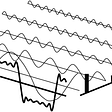## irreversible theory## What Do You Mean I Need the Median?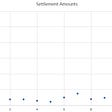## AGI-The Last Human Invention-Part I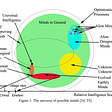## Prior and Likelihood Influence (a visualization)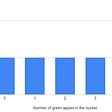## Little bit of Likelihoods## Bruno de Finetti and Exchangeability## Prim’s Algorithm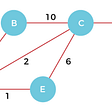## Vivekananda Das

Sharing ideas on Cause-and-Effect, Data Analysis in R, Stat Literacy, and Happiness | Ph.D. student @UW-Madison | More: https://vivekanandadas.com

## Checking your linear regression assumptions and how to check them## Non-Parametric Test in Statistics